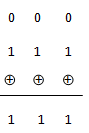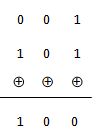# Interview Questions and Answers on Error Control Coding

### Q.1. What are the type of error control methods ?

Ans. Generally, the error control methods are of two types :

1. Error detection with retransmission
2. Forward acting error correction

In case of first method, when an error is detected, the retransmission request (ARQ) is sent back to the transmitter.

In the second method, the errors are detected and corrected by proper coding techniques at the receiver end. Whenever a single source transmits data to a number of receivers, the forward acting error correction is used. This is due to the fact that the retransmission is impossible in these cases.

### Q.2. Define the following terms :

(i) Code word                (ii) Block length

(iii) Code rate                (iv) Channel data rate

(v) code vectors            (vi) Hamming distance

(vii) Minimum distance

Ans.

(i) Code word : The encoded block of ‘n’ bits is known as a code word. It consists of message bits and redundant bits.

(ii) Block length : The number of bits ‘n’ after coding is known as the block length of the code.

(iii) Code rate : The ratio of message bits (k) and the encoder output bits (n) is known as code rate. Usually, code rate is denoted by ‘r’ i.e.,

r = k/n

we find that     0 < r < 1 .

(iv) Channel data rate : Channel data rate is the bit rate at the output of encoder. If the bit rate at the input of encoder is  Rs, then channel data rate will be,

Channel data rate (R0) =(k / n )× Rs

(v) Code Vectors : As ‘n’ bit code word can be visualized in an n-dimensional space as a vector whose elements or coordinates are the bits in the code word.

(vi) Hamming distance : The Hamming distance between the two code vectors is equal to the number of elements in which they differ.

As an example, let X = 101 and Y = 110. Since the two code vectors differ in second and third bits, therefore, Hamming distance between X and Y is ‘two’.

Hamming  distance is denoted by d(X,Y) or simply ‘d’. i.e.,

d(X,Y) = d = 2

Hence, it may be observed that the Hamming distance between (100) and (011) is maximum, i.e. 3 .

(vii) Minimum distance : Minimum distance ( dmin) is the smallest Hamming distance between the valid code vectors.

### Q.3. What are block codes ? Explain .

Ans. For the block of k message bits, (n-k) parity bits or check bits are added. This means that the total bits at the output of channel encoder are ‘n’. Such types of codes are known as (n,k) block codes.

### Q.4. Explain about Error detection and correction capabilities of Hamming codes .

Ans. Because the maximum distance ( dmin ) of Hamming code is 3, it can be used to detect double errors or correct single error.

For detecting double(2) errors =>  dmin ≥ 2+1  i.e.  dmin ≥ 3

and for correcting upto one (1) error =>  dmin ≥ 2(1) +1 i.e.  dmin ≥ 3

### Q.5. What are Repeated codes ? Explain .

Ans. In this code, only a single message bit is transmitted and q = 2t bits are parity bits. Hence k = 1.

Then the transmitted bits are,

n = 2t +1

This code is known as repeated code because many redundant check bits are transmitted along with a single message bit. This code can correct ‘t’ errors per block because this code uses many redundant check bits, therefore it requires a larger bandwidth .

Ans.

1. The error correcting and detecting methods of cyclic codes are simpler and easy to implement. These methods eliminate the storage needed for lookup table decoding. Therefore, the codes become powerful and efficient.
2. The encoders and decoders for cyclic codes are simpler compared to noncyclic codes.
3. Cyclic codes also detect error burst that span many successive bits.

1. The error detection in cyclic codes is simpler but error correction is little complicated since the combinational logic circuits in error detector are complex.

### Q.7. Show that C = { 000,111} is a linear code and C = { 000, 001, 101} is not a linear code.

Ans. The linear codes have an important property that any two code words of a linear code can be added in modulo-2 adder to produce a third code word in the code. Non-linear codes do not exhibit such a property. All the practically used codes are linear codes .

Properties of a linear code : A linear code should have the following properties :

1. The sum of code words belonging to the code is also a code word belonging to the code.
2. The all zero word is always a code word.
3. The minimum Hamming distance between two code words of a linear code is equal to the minimum weight of any non-zero code word.
4. The difference between two code words will yield a valid code word.

Note that even though the all zero code word is valid for a code, it does not guarantee that the code is linear code. the presence of an all zero code word is thus a necessary but not a sufficient condition for linearity.

Now consider the first code.

C ={ 000, 111}

Modulo-2 addition of the two code words is as followsThe results of this addition is a valid code word 111. Also the all zero code word 000 is present. Hence , the first code is linear code.

Now consider the second code.

C = { 000,   001,   101 }

Add the last two code word as follows :The result of this addition is not valid code word. therefore, the second code is not a linear code word .